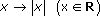## Study another free course

There are more than 800 courses on OpenLearn for you to choose from on a range of subjects.

Find out mo
Author(s): The Open University

Figure 19 shows a histogram of chest measurements (in inches) of a sample of 5732 Scottish soldiers.

[Image_Link]https://www.open.edu/openlearn/ocw/pluginfile.php/89953/mod_oucontent/oucontent/747/959931d9/6823ad53
Author(s): The Open University

## Question 1

Find the unknown angles in each of these diagrams, which represent part of the bracing structure supporting a marquee.

[Image_Link]https://www.
Author(s): The Open University

The sum of the angles of any triangle is 180°. This property can be demonstrated in several ways. One way is to draw a triangle on a piece of paper, mark each angle with a different symbol, and then cut out the angles and arrange them side by side, touching one another as illustrated.Author(s): The Open University

## Question 1

Draw a line of symmetry on each of the shapes below.

There is another kind of symmetry which is often used in designs. It can be seen, for instance, in a car wheel trim.Look at the trim on the left. It does not have line symmetry but
Author(s): The Open University

Look at the shapes below. The symmetry of the shape on the left and its relationship to the shape on the right can be thought of in two ways:

• Fold the left-hand shape along the central line. Then one side lies exactly on top of the other, and gives the shape on the right.

• Imagine a mirror placed along the central dotted line. The reflection in the mirror gives the other half of the shape.

Author(s): The Open University

Drawing circles freehand often produces very uncircle-like shapes! If you need a reasonable circle, you could draw round a circular object, but if you need to draw an accurate circle with a particular radius, you will need a pair of compasses and a ruler. Using the ruler, set the distance between the point of the compasses and the tip of the pencil at the desired radius; place the point on the paper at the position where you want the centre of the circle to be and carefully rotate the compass
Author(s): The Open UniversityA quadrilateral is a shape with four straight sides.
Author(s): The Open University

This section deals with the simplest geometric shapes and their symmetries. All of the shapes are two-dimensional – hence they can be drawn accurately on paper.

Simple geometric shapes are studied in mathematics partly because they are used in thousands of practical applications. For instance, triangles occur in bridges, pylons and, more mundanely, in folding chairs; rectangles occur in windows, cinema screens and sheets of paper; while circles are an essential part of wheels, gears a
Author(s): The Open University

## Question 1

Find α and β in each of the diagrams below.

[Image_Link]https://www.open.edu/openlearn/ocw/pluginfile.php/94675/mod_oucontent/
Author(s): The Open University

Other pairs of equal angles can be identified in Example 5. These pairs of angles occur in a Z-shape, as indicated by the solid line in the diagram below. Such angles are called alternate angles.

[Image_Link]https://www.open.edu/openlearn/o
Author(s): The Open University

Look at the line l, which cuts two parallel lines m and n.If you trace the lines at one of the intersections in the diagram below and place them over the lines at the other interse
Author(s): The Open University

Two straight lines that do not intersect, no matter how far they are extended, are said to be parallel. Arrows are used to indicate parallel lines.Author(s): The Open University

## Question 1

Find all the remaining angles in each of the diagrams below.

[Image_Link]https://www.open.edu/openlearn/ocw/pluginfile.php/94675/mod_oucontent/
Author(s): The Open University

When two straight lines cross, they form four angles. In the diagram below, these angles are labelled α, β, θ and φ and referred to as alpha, beta, theta and phi. The angles opposite each other are equal. They are called vertically opposite angles. Here α and β are a pair of vertically opposite angles, as are θ and φ. Although such angles are called ‘vertically opposite’, they do not need to be vertically above and bel
Author(s): The Open University

## Question 1

A company carried out a survey, recording how staff in a particular office spent their working time. The table shows the average number of minutes spent in each hour on various activities.

Author(s): The Open University

## Question 1

Calculate all the angles at the centres of these objects.

The modulus function provides us with a measure of distance that turns the set of complex numbers into a metric space in much the same way as does the modulus function defined on R. From the point of view of analysis the importance of this is that we can talk of the closeness of two complex numbers. We can then define the limit of a sequence of complex numbers in a way which is almost identical to the definition of the limit of a real sequence. Another analogue of real analysis arises
Author(s): The Open University

You will no doubt recall that in real analysis extensive use is made of the modulus function. It gives us a way of measuring the “closeness” of two numbers, which we exploit in writing expressi
Author(s): The Open University